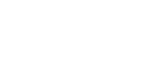ISSN 2542–0380 Труды Института механики им. Р.Р. Мавлютова Электронный научный журнал | Electronic Scientific Journal Proceedings of the Mavlyutov Institute of Mechanics© Институт механики
им. Р.Р. Мавлютова
УФИЦ РАНNasibullaeva E.Sh. Investigation of scattering from soundproof single sphere under external influence. Proceedings of the Mavlyutov Institute of Mechanics. 2017. 12(1). 73–82.
2017. Vol. 12. Issue 1, Pp. 73–82
URL: http://proc.uimech.org/uim2017.1.011,en
DOI: 10.21662/uim2017.1.011
Investigation of scattering from soundproof single sphere under external influence
Nasibullaeva E.Sh.
Mavlutov Institute of Mechanics, Ufa

### Abstract

The scattering from a single soundproof sphere is investigated during the passage of two types of waves: a spherical wave from a monopole radiation source and a plane wave. In solving the Helmholtz equation, a numerical technique based on the fast method of multipoles is used, which allows achieving high accuracy of the results obtained, and also minimizing computer time. The calculations are compared with known experimental data and a good agreement is obtained. Formulas are obtained for the main characteristic of the scattering field (the total scattering cross-section) for a sphere with an arbitrary acoustic impedance. Its dependence on the main parameters of the ”sphere – environment“ system for two kinds of the incident field is shown. A numerical parametric analysis of the value of the potential on the surface of a sphere for different values of its radius, complex impedance, the type of external influence, etc.

Keywords

scattering,
monopole radiation source,
plane wave,
single sphere,
total scattering cross-section

## Article outline

Purpose: generalization of the numerical model to determine the field around a single soundproof spheres with different values of the complex impedance by passing the two types of waves: a spherical wave from a monopole source and a plane wave.

Methodology: in solving the Helmholtz equation using a numerical technique based on the fast multipole method.

The findings of research:

1. the technique of the multipole expansion for an arbitrary acoustic impedance sphere is presented. The expansion formulas for two particular cases of an external field (a monopole radiation source and a plane wave) are obtained;

2. a comparison of the calculation results of this work with known experimental data have been made and gave good agreement;

3. formulas are obtained for the total scattering cross section for a sphere with an arbitrary acoustic impedance. The dependences of this characteristic on the main parameters of the system, such as the sphere radius and the complex impedance of the sphere, are shown for two types of the incident field;

4. a numerical parametric analysis of the value of the potential on the surface of the sphere for various values of its radius, complex impedance, and the type of external influence is carried out. A relationship is shown between the obtained results and the total cross section for scattering by the example on a rigid sphere.

Value: The obtained results will subsequently be generalized to the case of acoustic scattering from a sound-permeable sphere, i.e. sphere through which surface the wave passes (the cases of liquid droplets or air bubble), and the scattering from many spheres.